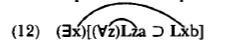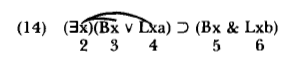# 3.2: Quantifier Scope, Bound Variables, and Free Variables

In the last example we saw that the variable at 3 is bound by the quantifier at 1 and the variable at 4 is bound by the quantifier at 2. This case contrasts with that of a variable which is not bound by any quantifier, for exampleIn (8), the occurrence of 'x' at 3 is bound by the quantifier at 2. However, the occurrence of 'x' at 1 is not bound by any quantifier. Logicians say that the occurrence of 'x' at 1 is Free. In (9), the occurrence of 'x' at 3 is free because the quantifier at 1 binds only variables in the shortest full sentence which follows it. Logicians call the shortest full sentence following a quantifier the quantifier's Scope. In (9), the 'x' at 3 is not in the scope of the quantifier at 1. Consequently, the quantifier does not bind 'x' at 3.

All the important ideas of this section have now been presented. We need these ideas to understand clearly how to apply the methods of derivations and truth trees when quantifiers get stacked on top of each other. All we need do to complete the job is to give the ideas an exact statement and make sure you know how to apply them in more complicated situations.

Everything can be stated in terms of the simple idea of scope. A quantifier is a connective. We use a quantifier to build longer sentences out of shorter ones. In building up sentences, a quantifier works just like the negation sign: It apples to the shortest full sentence which follows it. This shortest full following sentence is the quantifier's scope:

Definition: scope

The Scope of a quantifier is the shortest full sentence which follows it. Everything inside this shortest full following sentence is said to be in the scope of the quantifier.

We can now define 'bound' and 'free' in terms of scope:

• A variable, u, is Bound just in case it occurs in the scope of a quantifier, (Vu) or (Ǝu).
• A variable, u, is Free just in case it is not bound; that is, just in case it does not occur in the scope of any quantifier, (Vu) or (Ǝu).

Clearly, a variable gets bound only by using a quantifier expressed with the same variable. 'x' can never be bound by quantifiers such as '(Vy)' or '(Ǝz)'.

Occasionally, students ask about the variables that occur within the quantifiers-the 'x' in '(Ǝx)' and in '(Vx)'. Are they bound? Are they free? The answer to this question is merely a matter of convention on which nothing important turns. I think the most sensible thing to say is that the variable within a quantifier is part of the quantifier symbol and so does not count as either bound or free. Only variables outside a quantifier can be either bound or free. Some logicians prefer to deal with this question by defining the scope of a quantifier to include the quantifier itself as well as the shortest full sentence which follows it. On this convention one would say that a variable within a quantifier always binds itself.

These definitions leave one fine point unclear. What happens if the variable u is in the scope of two quantifiers that use u? For example, consider

(10) (Ǝx)[(Vx)Lxa ⊃ Lxb]
1 234

The occurrence of 'x' at 3 is in the scope of both the 'x' quantifiers. Which quantifier binds 'x' at 3?

To get straight about this, think through how we build (10) up from atomic constituents. We start with the atomic sentences 'Lxa' and 'Lxb'. Because atomic sentences have no quantifiers, 'x' is free in both of these atomic sentences. Next we apply '(Vx)' to 'Lxa', forming '(Vx)Lxa', which we use as the antecedent in the conditional

(11) (Vx)Lxa ⊃ Lxb
23 4

In (11), the occurrence of 'x' at 3 is bound by the quantifier at 2. The occurrence of 'x' at 4 is free in (11).

Finally, we can clearly describe the effect of '(Ǝx)' when we apply it to (11). '(Ǝx)' binds just the free occurrences of 'x' in (11). The occurrence at 4 is free and so gets bound by the new quantifier. The occurrence at 3 is already bound, so the new quantifier can't touch it. The following diagram describes n the overall effect:First, the occurrence at 3 is bound by the quantifier at 2. Then the occurrence at 4 is bound by the quantifier at 1. The job being done by the 2-3 link is completely independent of the job being done by the 1-4 link.
Let's give a general statement to the facts we have uncovered:

A quantifier (Vu) or (Ǝu) binds all and only all free occurrences of u in its scope. Such a quantifier does not bind an occurrence of u in its scope which is already bound by some other quantifier in its scope.

We can make any given case even clearer by using different variables where we have occurrences of a variable bound by different quantifiers. So, for example, (10) is equivalent toIn (12), there can be no confusion about which quantifier binds which variable-we keep track of everything by using different variables. Why, then, didn't we just resolve to use different variables from the beginning and save ourselves a lot of trouble? We could have done that, but then the definition of the sentences of predicate logic would have been much more complicated. Either way, we have work to do. Besides, the formulation I have presented here is the one traditionally used by logicians and so the one you will need to know if you study more logic.

Let's look at another, slightly more complicated, example to make sure you have put this all together. Draw in the lines which show which quantifier binds which variable in the following:

(13) (Ǝx)[(Ǝx)(Bx v Lxa) ⊃ (Bx & Lxb)]

If you are having trouble, think through how (13) gets built up from its parts. Inthe quantifier at 2 applies only to the shortest full sentence which follows it, which ends before the '⊃'. So the occurrences of 'x' at 3 and 4 are both bound by the quantifier at 2. The two occurrences of 'x' at 5 and 6 are not in the scope of a quantifier and are both free. So when we apply the second '(Ǝx)' to all of (14), the new '(Ǝx)' binds only the 'x's which are still free in (14), namely, the 'x's which occur at 5 and 6. In sum, the pattern of binding isWe can make this pattern even clearer by writing the sentence equivalent to (13):

(15) (Ǝx)[(Ǝz)(Bz v Lza) ⊃ (Bx & Lxb)]

In practice, of course, it is much better to use sentences such as (15) and (12) instead of the equivalent (13.) and (lo), which are more difficult to interpret.

Exercise

3-1. In the following sentences draw link lines to show which quantifiers bind which variables and say which occurrences of the variables are bound and which are free:

a) L z z b) (Vy)(Vz)L z y c) (Vz)(Bz ⊃ L x z)
1 21 2 12 3

d) (Ǝx)[Lxz & (Vy)(Lxy v Lzx)]
12 34 56

e) (Vx)(Lyx ⊃ (Bx ⊃ (Ǝx)Lyx)]
12 3 45